1.3 Cubes and Cube roots:

 In the previous classes we had studied an object called cuboid which has three dimensions  namely length, breadth and height. Cube is special figure whose length=breadth=height Size(volume) of a cube = length*length*length = (length)3 if a = 1,2,3  units, then the volume of cube respectively is =  1*1*1 = 1,  2*2*2=8, 3*3*3=27  units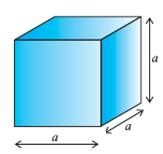Is there a relationship between the numbers 1, 8, 27, 64and  1, 2, 3, 4… ?

They are numbers obtained by multiplying the same number thrice from the 2nd set of numbers.

Cube numbers are integers raised to the power of 3. They are of the form n3.

If n3 is the cube of number n then n is the ‘cube root’ of the number n3 .Cube root is denoted by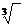So 1,2,3,4 are cube roots of the numbers 1, 8, 27, 64 respectively.

Definition: Any number which is a product of three identical numbers is called ‘cube’.

The volume of a solid figure of three dimension=length*breadth*depth(we learn this in Geometry).

Cube is a solid figure whose length, breadth and depths are same.The volume of a cube = length*length*length = (length)3

1.3 Problem 1 : If the side of a cube is 7cms find its volume

Solution:

Volume of a cube = (length)3Volume of cube of side 7cms length= (7)3

=7*7*7 = 343 cubic cms

1.3.1 Finding cube root by factorisation

In this method we find all prime factors of the given number and then group the common factors in triples, such that all two factors in each pair are the same. If some factors do not appear in triples then the number is not a perfect cube and we stop the process of grouping.

1.3.1 Problem 1 : Find the cube root of 42875

Solution:

The factors of 42875 are  5,5,5,7,7,742875 = 5*5*5*7*7*7 = 53*33= (5*7)3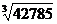= 5*7 =35

1.3.1 Problem 2: Find the least number by which 432 must be multiplied or divided to make it a perfect cube.

Solution:

The factors of 432  are  3,3,3,4.4 = (3)3*(4)2

We note that the factor 4 appears only 2 times.

1. If we multiply 432 by 4 we get 432*4 = (3)3*(4)3= (3*4)3, so when the smallest number 4 multiplies 432, it gives us the cube number (=1728=123)

2. If we divide 432 by 16 we get 432/16= 33, so when the smallest number 16 divides 432, it gives us the cube number (=27 = 33).

1.3.1 Problem 3: If 1 cubic cc of water weighs 1 gram, what is the weight of 1 cubic meter of water?

Solution:

 Volume of cube of side of 1meter (Note: 1m=100cm) = side*side*side= (side)3cc = (100)3cc = 1000000cc Since 1 cc of water weighs one gram 1000000cc of water will weigh 1000000gm = 1000 kg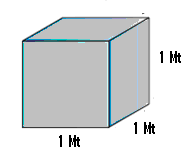1.3 Summary of learning

 No Points studied 1 Cubes , finding cube root by factorisation method

Properties of perfect cubes and cube roots:

1.      The relationship between unit’s digit of cube and its cube root is as follows:

 Unit’s digits of cube       ==è 0,1,4,5,6,9 2 8 3 7 Unit’s digit of cube root  ==è Same as that of unit’s digits of cube 8 2 7 3

2.  If a number is negative, its cube is also negative ((-7)3 = -343: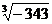= -7)

3.  Cube of an even number is even and cube of an odd number is odd

4.  Cube root of a rational number = cube root of numerator/cube root of denominator (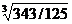=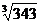/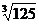= 7/5)